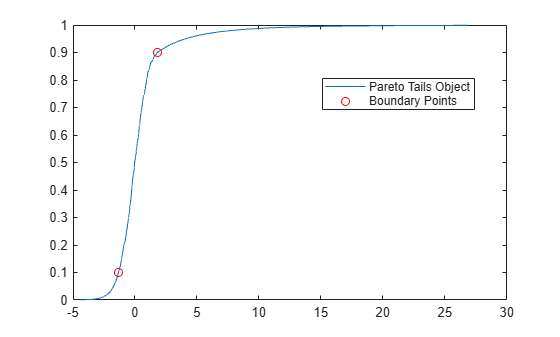# boundary

Piecewise distribution boundaries

## Syntax

``[p,q] = boundary(pd)``
``[p,q] = boundary(pd,j)``

## Description

example

````[p,q] = boundary(pd)` returns the boundary points between segments in `pd`, the piecewise distribution. `p` is a vector of the cumulative probabilities at the boundaries, and `q` is a vector of the corresponding quantiles.```
````[p,q] = boundary(pd,j)` returns boundary values of the `j`th boundary.```

## Examples

collapse all

Generate a sample data set and create a `paretotails` object by fitting a piecewise distribution with Pareto tails to the generated data. Find the boundary points between segments in a `paretotails` object by using the object function `boundary`.

Generate a sample data set containing 20% outliers.

```rng('default'); % For reproducibility left_tail = -exprnd(1,100,1); right_tail = exprnd(5,100,1); center = randn(800,1); x = [left_tail;center;right_tail];```

Create a `paretotails` object by fitting a piecewise distribution to `x`. Specify the boundaries of the tails using the lower and upper tail cumulative probabilities so that a fitted object consists of the empirical distribution for the middle 80% of the data set and generalized Pareto distributions (GPDs) for the lower and upper 10% of the data set.

`pd = paretotails(x,0.1,0.9)`
```pd = Piecewise distribution with 3 segments -Inf < x < -1.33251 (0 < p < 0.1): lower tail, GPD(-0.0063504,0.567017) -1.33251 < x < 1.80149 (0.1 < p < 0.9): interpolated empirical cdf 1.80149 < x < Inf (0.9 < p < 1): upper tail, GPD(0.24874,3.00974) ```

Return the boundary values between the piecewise segments by using the `boundary` function.

`[p,q] = boundary(pd)`
```p = 2×1 0.1000 0.9000 ```
```q = 2×1 -1.3325 1.8015 ```

The values in `p` are the cumulative probabilities at the boundaries, and the values in `q` are the corresponding quantiles.

Plot the cdf of the `paretotails` object and mark the boundary points on the figure.

```xi = sort(x); plot(xi,cdf(pd,xi)) hold on plot(q,p,'ro') legend('Pareto Tails Object','Boundary Points','Location','best') hold off```## Input Arguments

collapse all

Piecewise distribution with Pareto tails, specified as a `paretotails` object.

Boundary index indicating which boundary to return, specified as a positive integer.

Data Types: `single` | `double`

## Output Arguments

collapse all

Cumulative probability at each boundary, returned as a numeric vector of range `(0,1)` values.

Quantile at each boundary, returned as a numeric vector.

Introduced in R2007a